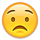## Forum Stats

• 3,855,570 Users
• 2,264,523 Discussions

Discussions

# Applets on a tripod page

I'm trying to get a really simple Applet to work on my lycos / tripod homepage. It doesn't seem to work. I haven't published any Applets before, so I might be missing something obvious. Can someone give me some pointers.

Info:
Its a single class JApplet thing.
Doesn't do anything complicated.
I get a security exception when I run from the tripod site although it runs fine on my own machine.

This is the top line exception I get

Tripod won't help meSo can you .....

Cheers,
Dave.

• Probablr not a simple Applet , can we see the code ?
• Here is the code, just asks you some questions and gives you diff answers depending on score. Pretty simple I'd say.
```import java.awt.*;
import java.applet.*;
import javax.swing.*;
import java.awt.event.*;
import java.awt.color.*;

public class Hosometer extends JApplet {

int total;
int question;
JTextArea q;

JButton one;
JButton two;
JButton three;
JButton four;
JButton five;

String [] questions = { "Q1",
"Q2",
"Q3",
"Q4",
"Q5",
"Q6",
"Q7"};

public void init() {
setBackground(new Color(5866895));
total = 0;
q = new JTextArea(7,30);
q.setEditable(false);
q.setBackground(new Color(5866895));
one = new JButton("1 (bad)");
public void actionPerformed(ActionEvent e) {
next(1);
}
});

two = new JButton("2");
public void actionPerformed(ActionEvent e) {
next(2);
}
});

three = new JButton("3(moderate)");
public void actionPerformed(ActionEvent e) {
next(3);
}
});
four = new JButton("4");
public void actionPerformed(ActionEvent e) {
next(4);
}
});

five = new JButton("5 (good)");
public void actionPerformed(ActionEvent e) {
next(5);
}
});

JPanel bt = new JPanel();
JPanel main = new JPanel();
bt.setBackground(new Color(5866895));
main.setBackground(new Color(5866895));

q.setText(questions);

}

public void next(int score)
{
total = total + score;
question ++;
if(question<questions.length)
q.setText(questions[question]);
else{

one.setEnabled(false);
two.setEnabled(false);
three.setEnabled(false);
four.setEnabled(false);
five.setEnabled(false);
int pct = (total * 100) / (35);
if(total < 8)
q.setText("");
else if (total < 16)
q.setText("");
else if (total < 22)
q.setText("");
else if (total < 28)
q.setText("");
else if (total < 32)
q.setText("");
else if (total < 35)
q.setText("");
else if (total == 35)
}  }

}```
• I have problems pasting the code can you email it to me

[email protected]
• This look's good:
``` import java.awt.*;
import java.applet.*;
import java.awt.event.*;
import javax.swing.*;

public class Hosometer extends JApplet implements ActionListener
{
int      total=0, question;
JButton   one, two, three, four, five;
JTextArea q;
String[]  questions = { "Q1",
"Q2",
"Q3",
"Q4",
"Q5",
"Q6",
"Q7"};

public Hosometer()
{
q = new JTextArea(7,30);
q.setEditable(false);

one = new JButton("1 (bad)");
two = new JButton("2");
three = new JButton("3(moderate)");
four = new JButton("4");
five = new JButton("5 (good)");

JPanel bt   = new JPanel();
JPanel main = new JPanel();
bt.setBackground(new Color(5866895));
main.setBackground(bt.getBackground());

q.setText(questions);
setContentPane(main);

}
public void init()
{
}
public void actionPerformed(ActionEvent e)
{
if (e.getSource() == one)   next(1);
if (e.getSource() == two)   next(2);
if (e.getSource() == three) next(3);
if (e.getSource() == four)  next(4);
if (e.getSource() == five)  next(5);
}

public void next(int score)
{
total = total + score;
question ++;
if (question < questions.length)
q.setText(questions[question]);
else
{
one.setEnabled(false);
two.setEnabled(false);
three.setEnabled(false);
four.setEnabled(false);
five.setEnabled(false);
int pct = (total * 100) / (35);
if (total < 8)       	  q.setText("Answer1");
else if (total < 16)  q.setText("A2");
else if (total < 22)  q.setText("A3");
else if (total < 28)  q.setText("A4");
else if (total < 32)  q.setText("A5");
else if (total < 35)  q.setText("A6");
else if (total == 35) q.setText("Answer");
}
}
}```
Noah
• Excellent seem to have it working now.
Took the codebase line out of the HTML and it works.

Thanks for the help with the code as well.

Dave.
This discussion has been closed.